### Home > PC > Chapter 2 > Lesson 2.3.5 > Problem2-128

2-128.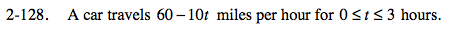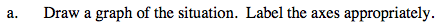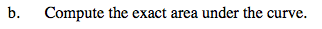Break the shape into a rectangle and a triangle and sum the two areas. Or, use the formula for the area of a trapezoid.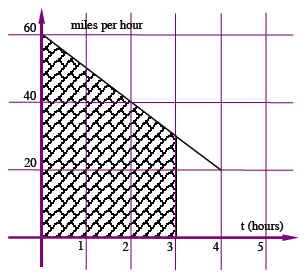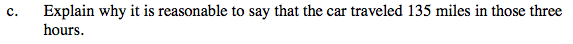It is the units on the x and y axis. Area is length times width.

$\frac{\text{miles}}{\text{hour}}\cdot \text{hours}$